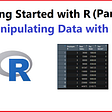# Necessary First Steps

`library(shiny)library(dplyr)library(ggplot2) NL_CY <- read.csv("NL_CY.csv")`

# Building the User Interface

`ui <- fluidPage(  titlePanel(),   sidebarLayout(    sidebarPanel(),    mainPanel()  ))`
`sidebarPanel(    selectInput(        inputId = "PitcherInput",         label = "Select Pitcher",         choices = sort(unique(NL_CY\$player_name))),    dateRangeInput(        inputId = "DateRangeInput",         label = "Select Date Range",         start = min(NL_CY\$game_date),         end = max(NL_CY\$game_date)),    img(src = "ss_logo.png",         style = "display: block; margin-left: auto;                  margin-right: auto;",         height = 150,         width = 150))`
`mainPanel(    tabsetPanel(        tabPanel("Pitch Usage - Bar Chart", br(),                  plotOutput("barchart")),        tabPanel("Pitch Velocity - Box Plot", br(),                  plotOutput("boxplot")),        tabPanel("Pitch Velocity Trend - Line Plot", br(),                  plotOutput("lineplot"))    ))`

# Writing Server Code

`server <- function(input, output) {  output\$plot1 <- renderPlot({    dataFilter <- reactive({})    ggplot()  })    }`
`output\$barchart <- renderPlot({    dataFilter <- reactive({        NL_CY %>%             filter(player_name == input\$PitcherInput,                   between(game_date, input\$DateRangeInput,                                      input\$DateRangeInput)) %>%            group_by(pitch_name) %>%            summarize('count' = n())    })    ggplot(dataFilter(),            aes(x = reorder(pitch_name, -count),                y = count,                fill = pitch_name)) +         geom_bar(stat = "identity") +        labs(x = "Pitch Type",              y = "Count",              title = "Pitch Usage") +        theme_bw() +         theme(legend.position = "none",               plot.title = element_text(hjust = 0.5,                                         face = "bold",                                         size = 16)) +        theme(axis.title = element_text(size = 14),               axis.text = element_text(size = 12))}, width = 850, height = 450)`

# Tying It All Together

`shinyApp(ui = ui, server = server)`

--

--

--

## More from Sam Bornstein

Driveline Baseball Operations Analyst

Love podcasts or audiobooks? Learn on the go with our new app.

## How to use Anaconda on a mac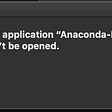## Python, and the Story of Recursion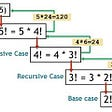## CICD with Bitbucket Pipeline## Intel Arc Alchemist Gaming Graphics Card Pictured, Gets Detailed Cooler & PCB Shots## Sam Bornstein

Driveline Baseball Operations Analyst

## Bar, scatter, line, and box-plot in R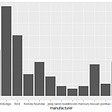## The Data Sandbox | Making the Connection with Crosstalk## K-Means Clustering in R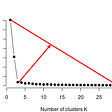## Manipulating Data with R